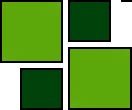ISSN 1842-4562 Member of DOAJ

# Using Structural Equation and Item Response Models to Assess Relationship between Latent Traits

## Abstract

We deepen the two main approaches to the problem of measurement error in social sciences, the Structural Equation Models (SEM) and the Item Response Theory Models (IRM), comparing two different estimation procedures. The One-step procedure (related to SEM) requires that researcher specifies a complete model of both measurement aspects (single link between the latent variable and its indicators) and structural aspects (links between different latent variables), with the model parameters estimated simultaneously. In the Two-step procedure (related to IRM), we first estimate the measures (one for each construct), then we will assess, through a regression model, the relationships between these measures and the latent variables that they represent. Our aim is to define a Two-step method that, using information obtained in the first step about the measurement error, presents low levels of bias and loss of efficiency, as close as possible to that of One-step method.

(top)